Beware of recent phishing e-mails. Use our official contact addresses only.
413-284-9975

# Note 74: Examination of Source Design in Electrospray-TOF Using SIMION 3D

By Steven M. Colby

Presented at EAS, Summerset, NJ., November 1998

INTRODUCTION

The propose of this poster is to demonstrate that methods used to improve the resolution of MALDI-TOF instruments can be extended to other TOF instruments. Specifically, we examine the calculation of instrument conditions in an electrospray-TOF instrument. Instrument performance is simulated using SIMION 3D ion optics software.

A review is given of the methods for calculating instrument conditions in time of flight instruments. This includes: 1. the calculation of expected time of flight; 2. the calculation of space focusing conditions; and 3. the calculation of improved conditions for MALDI instruments. We then demonstrate how similar calculations can be made to determine improved conditions for an electrospray-TOF geometry. Finally, simulated results are shown and discussed.

Calculation of Ion Time of Flight

The flight time of an ion can be calculated from the following fundamental equations: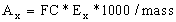Where Ax is the acceleration in region x in units of meters/sec2, FC is the Faraday Constant, Ex is the electric field in region x (Volts/meter), and mass is the ion's mass in Daltons.

Velocity (Vx) at the end of an acceleration over a distance of length Dx is given by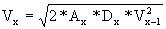Where Vx-1 is the velocity prior to the effects of Ax.

The time (Tx) spent in an acceleration region is given by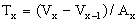The time spent in a field free region is given by Tx = Dx/Vx and the total time of flight (Ttotal )is the sum of the times spent in each region.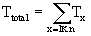Calculation of Space Focusing Conditions

Space focusing conditions are intended to minimize the dependence of Ttotal on the initial ion position. This position is typically referred to as X0. In the above notation X0 can be defined relative to the end of the first acceleration region and thus X0 = D1.

In order to find space focusing conditions, as defined by Wiley and McLaren (1), one first assumes that the average initial velocity (V0) is equal to zero. The derivative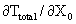is then set equal to zero and one can solve for a ratio of the first two acceleration regions (E1/E2). (In a linear instrument with a two step acceleration source followed by a drift region). By selecting a drift energy in the field free region, the voltages to be applied in the source can then be determined. Note that for instrument geometries where the average initial velocity is not equal to zero, as MALDI-TOF instruments, space focusing calculations as describe by Wiley and McLaren do not provide the optimal answer.

Calculation of Focusing Conditions In a MALDI-TOF

In a MALDI-TOF instrument, where ions are desorbed towards the detector, the average initial velocity of ions does not equal zero. There are two approaches that may be taken to avoid the assumptions made by Wiley and McLaren (2,3). First, one could assume an average non-zero initial velocity. This has the drawback that initial velocities are known to vary widely. Thus, any single value would be a poor representative for all the ions. In an alternative approach one could use the relationship V0 = (D-X0)/t. Where D is the total length of the first acceleration region and t is a delay time between the desorption of the analytes and their acceleration toward the detector. This equation differs from that used by Wiley and McLaren, in their description of time lag focusing, in that it expresses a relationship between the velocity of an ion and its distance from the sample plate. In contrast, time-lag-focusing utilizes an equation that relates an ion's velocity with its displacement from an unknown origin. The above equation can, therefore, be used to correlate the spatial and velocity distributions at the time of extraction. By substituting it into the basic equation for time of flight discussed above, the V0 term can be eliminated. X0 then represents both the velocity and spatial distributions at the time of extraction. Optimal focusing conditions can now be found by taking the derivative to the time of flight with respect to X0. No further assumptions about V0 need to be made. In practice, other optimization routines (i.e. SIMPLEX optimization) are preferable since under the best conditions the time of flight as a function of X0 is no longer a simple parabola (2). Alternative optimization routines are simplified by the elimination of the independent V0 variable. They also permit one to optimize other parameters such as the delay time before acceleration.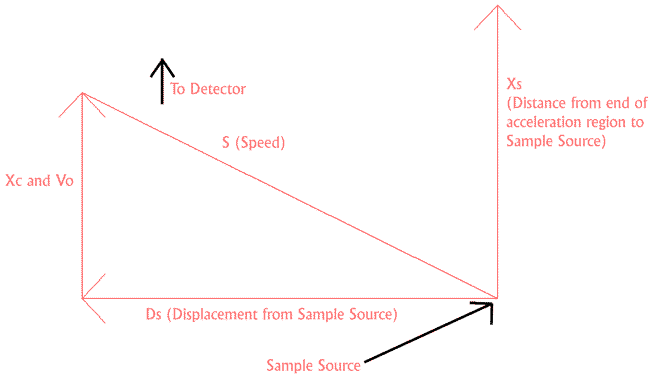Figure 1. Definition of Instrument Source Parameters

The above calculations have dramatically improved the performance of MALDI instruments since they were first introduced in 1994 (4,5,6). The same principles can be applied to other instrument geometries (3). These include systems in which the sample is desorbed from a surface parallel to the main axis of the flight tube and systems in which sample is continuously introduced in a direction perpendicular to the main axis of the flight tube (See Figure 1). This second geometry may be relevant to some electrospray-TOF systems. The remainder of this poster examines how improved focusing conditions may be found for systems with an electrospray type geometry. SIMION 3D software is used to estimate improvements in peak width and instrument resolution.

The Application of Correlation Principles To Instruments With an "Electrospray" TOF Geometry

Figure 1 illustrates the relevant geometry and defines the distance (X, D) and velocity (V) variables used below. It is assumed that the sample continuously enters the instrument from a point referred to as the sample source. A voltage pulse is periodically applied to the first acceleration region to extract ions toward the detector. The correlation equation for this geometry is not as simple as the equation used in the MALDI geometry. For this geometry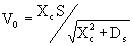Where X0 = Xs - Xc. Xs represents the displacement between the sample source and the end of the first acceleration region. Ds represents the displacement, perpendicular to Xs, between the sample source and the point of ionization. S represents the speed at which the sample leaves the sample source. We are assuming that S and Xs are the same for all molecules and we are only considering a two dimensional view of the source. We will also have to take into account the fact that that Ds will take a range of values. The above equations can be substituted into a basic equation for the time of flight of an ion. The resulting formula can then be used to calculate optimal instrument conditions.

Calculation of Optimal Instrument Conditions For the Electrospray-TOF Instrument

For the purposes of this poster we have calculated optimal conditions using an Excel spreadsheet. We assumed the patameters listed in Table 1. These conditions are intentionally different from any commercial instrument of which we are aware. Our purpose is to demonstrate calculation and simulation methods, not to find improved focusing conditions for any specific instrument.

Table 1

 Ds 3 ± 0.2 cm Xs 5 mm D1 1 cm D2 8 mm D3 (Field Free) 80 cm Drift Energy 5000 eV Ion Mass/Charge 5,000 Ion Speed 982 m/s

Optimal values were found for the two non-zero voltages in the instrument source. It was assumed that ions leaving the sample source at angles of greater than six degrees did not reach the extraction region. A calculation was also performed using the space focusing methods of Wiley and McLaren. These results were used for comparison. The results obtained are shown in Table 2 below.

Table 2

 Calculation Variable Value (Volts) Correlation First Voltage 5284.0 Second Voltage 4216.0 Space Focusing First Voltage 5257.12 Second Voltage 4742.88

Simulation of Calculated Results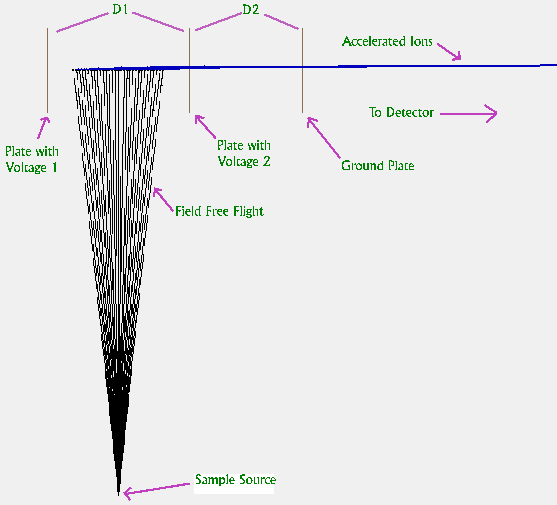Figure 2. Instrument Simulation In SIMION 3D Software

In order to demonstrate the improvement in peak width resulting from correlation conditions, simulations were performed using the ion optics software SIMION 3D (7). An illustration from this simulation is shown in Figure 2. Ions first appear from below and travel to the center of the instrument source. A voltage pulse is then applied and ions are rapidly accelerated toward the detector (not shown). To clarify the illustration, we have changed the color of the ion trajectory at the time the voltage pulse is applied. In the figure 30 ions are shown; however, in the simulation, two thousand ions were flown. They were given initial angles between ± six degrees and were extracted at five possible values of Ds between 28 and 32 mm.

Results.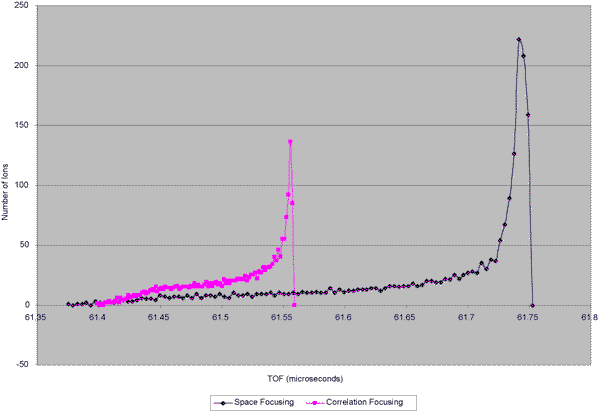Figure 3 Calculated Results

Ion times of flight were recorded and histograms were calculated in order to generate possible peak shapes. The resulting plots are shown in Figure 3. The simulated peak resulting from correlation conditions is substantially narrower than that resulting from Space Focusing conditions. These results predict a narrowing of experimental peaks by about 60%. This improvement is not as dramatic as that found in the MALDI geometry do to the various possible values of Ds. However, they do show a potential improvement to instrument resolution.

Conclusions

We have demonstrated methods in which improved instrument conditions may be calculated for a specific geometry of TOF instrument. These conditions have been simulated using SIMION 3D software. We have made significant assumptions in our calculations. For example, we have used a very simple instrument without items such as focusing elements between the sample source and the instrument source. We have also not included space charge effects, we have assumed that Xs is the same for all analytes, and we have not accounted for changes in velocity due to collisions or the desolvation process. These factors may require changes to the correlation equation and may effect the improvement that is possible in a real instrument.

References

1. Wiley, W. C.; McLaren I. H. Rev. Sci. Instrum. 1955, 26, 1150-1157.
2. Colby, S. M.; Reilly, J. P. Anal. Chem. 1996, 68, 1419-1428.
3. U.S. Patents 5,504,326; 5,510,613, and 5,712,479
4. Brown, R. S.; Lennon, J. J.; Christie, D. Desorption '94, Sunriver Lodge, OR, March 27-31, 1994, p. 63. and Lennon, J. J.; Brown, R. S. 42nd ASMS Conf. On Mass Spectrom. 1994, 501.
5. Colby, S. M.; King, T. B.; Reilly, J. P. Rapid Commun. Mass Spectrom. 1994, 8, 865-868.
6. Whittal, R. M.; Li, L. Anal. Chem. 1995, 67, 1950-1954.
7. SIMION 3D software by David Dahl. See www.sisweb.com.